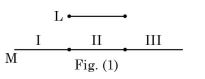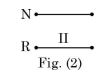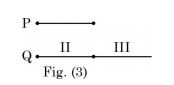The Eladr Protocol is a decentralized, security and efficiency enhanced Web3 noSQL database powered by IPFS as the data storage layer https://ipfs.io/, and the Cardano block chain as the rewards token platform, https://cardano.org/. It provides a JSON based, IPFS layer 2 solution for data indexing and retrieval in an 'append only' file system built with open source Node.js API libraries.

The ELADR token was designed to incentivize and reward community members as a proof of contribution. Token holders are also granted access to EduLadder.com premium features as well as associated ELADR token enabled apps.

WHITE PAPER Buy Now Try BETA

Real Problems! Real Experts!

Watch related videos of your favorite subject.
Connect with students from different parts of the world.
See Our team
Wondering how we keep quality?
Got unsolved questions?

GATE-ME2-2019-->View question

Taged users:

Likes:
Be first to like this question

Dislikes:
Be first to dislike this question
##### Correct Option: BFLM = F(I) + F(II) + F(III)
Where F is shape factor of surface area I, II and III of M with surface area of ‘L’
0.8 = F(I) + F(II) + F(III)
∵ (I) and (III) are symmetrical in shape so, shape factor of these surface will be same i.e. F(I) = F(III)
So, 0.8 = 2F(I) + F(II)... (1)In this area (II) is same as fig. (I) area II.
i.e. FNR = F(II) = 0.4... (2)
From equation (1),

 F(III) = 0.8 - 0.4 = 0.2 2Here, FPQ = F(II) + F(III)
= 0.4 + 0.2 = 0.6

Likes:
Be first to like this answer

Dislikes:
Be first to dislike this answer